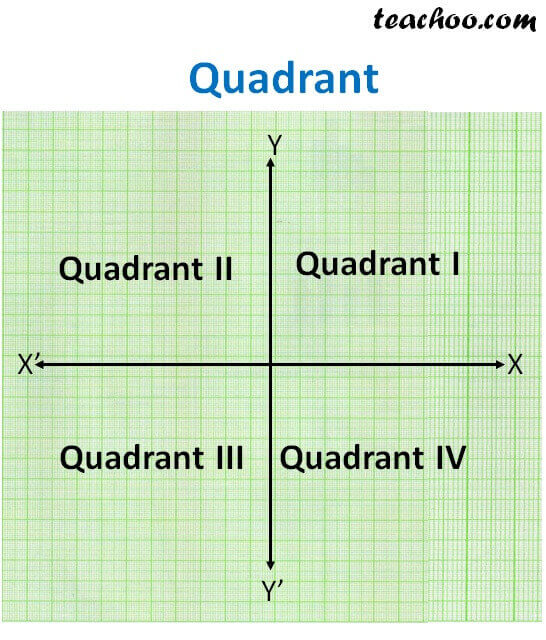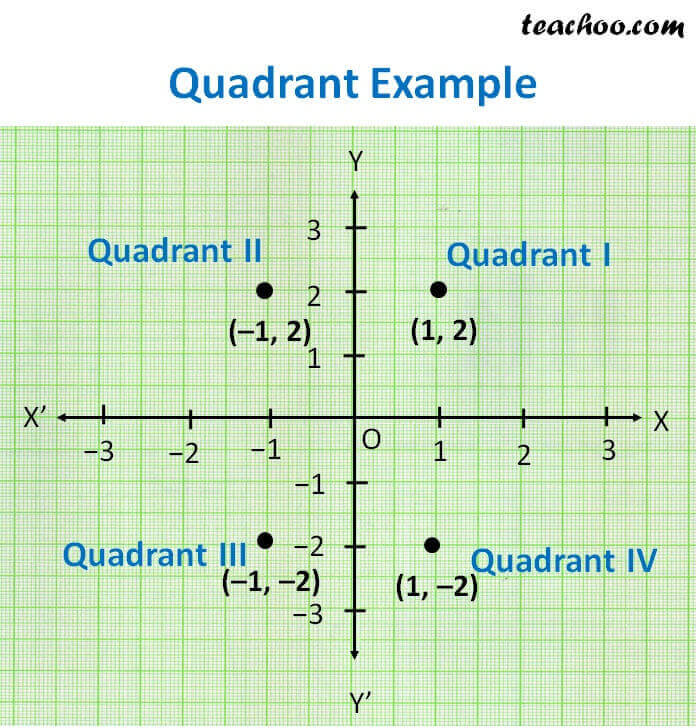Observing points on the graph

Chapter 3 Class 9 Coordinate Geometry
Concept wise

The x and y axis divide the graph into 4 quadrants

1. In 1st quadrant -  x is positive, y is positive

2. In 2nd quadrant – x is negative, y is positive

3. In 3rd quadrant – x is negative, y is negative

4. In 4th quadrant – x is positive, y is negative.Quadrant X Y Example I + + (1, 2) II − + (−1, 2) III − − (−1, −2) IV + − (1, −2)Get live Maths 1-on-1 Classs - Class 6 to 12